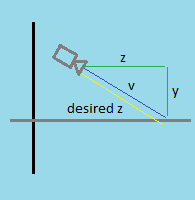# Movement relative to Camera and XZ Plane

I have a 3rd person controller set up based on the 3DBuzz’s tutorials.
I want to move in the direction the camera is facing but only using the input on the XZ plane. And so far i’ve come up with this:

``````    void ProcessMotion()
{
// Transform MoveVector to World Space
MoveVector = Camera.main.transform.TransformDirection(MoveVector);
MoveVector = new Vector3(MoveVector.x, 0.0f, MoveVector.z);

// Normalize MoveVector if magnitude > 1
if (MoveVector.magnitude > 1)
MoveVector = Vector3.Normalize(MoveVector);

// Move the Character in World Space
TPS_Controller.CharacterController.Move(MoveVector * moveSpeed * Time.deltaTime);
}
``````

The result of this is when i move, if i look up or down (rotate the camera on X axis), the character slows down because of the Z value received from the TransformDirection:I found a workaround for this issue, i don’t know if this is the most effective solution but it works 100%.
I created an empty game object which stores ONLY the Y rotation value of the camera. So the direction of this new game object is only on the XZ plane.

Code:

``````    void ProcessMotion()
{
// Transform MoveVector to World Space
MoveVector = Pivot.Instance.transform.TransformDirection(MoveVector);

// Normalize MoveVector if magnitude > 1
if (MoveVector.magnitude > 1)
MoveVector = Vector3.Normalize(MoveVector);

// Move the Character in World Space
TPS_Controller.CharacterController.Move(MoveVector * Time.deltaTime);
}
``````

New GameObject code:

``````public class Pivot : MonoBehaviour
{
public static Pivot Instance;

void Awake ()
{
Instance = this;
}

void Update ()
{
transform.rotation = new Quaternion(0.0f, Camera.main.transform.rotation.y, transform.rotation.z, Camera.main.transform.rotation.w);
}
}
``````